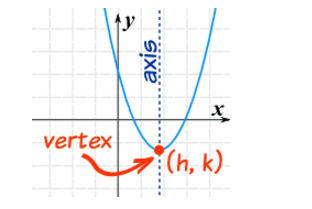# Range over some other range!Given $y=\frac{2x}{1+x^{2}}$, where $x$ and $y$ are real numbers, what is the range of $y^{2}+y-2$?

×